Tasneem

Arekere, Bangalore, India - 560076Tasneem

Arekere, Bangalore, India - 560076.

3.8

UrbanPro Rating

Details verified of Tasneem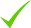IdentityEducation

Know how UrbanPro verifies Tutor details

Identity is verified based on matching the details uploaded by the Tutor with government databases.

Overview

I am a teacher in the oxford college of dental and my specialization is orthodontistry. i have a degree in BDS and my skills are proficient teaching

Languages Spoken

Hindi Proficient

Tamil Proficient

English Proficient

Education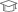the oxford college of dental 2010

Bachelor of Computer Science (B.Sc. (Computer Science))

Arekere, Bangalore, India - 560076

Verified Info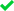Phone VerifiedEmail Verified

Demo Class

No

Report this Profile

Is this listing inaccurate or duplicate? Any other problem?

Please tell us about the problem and we will fix it.

Please describe the problem that you see in this page.

Type the letters as shown below *

Please enter the letters as show below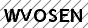Class 12 Tuition Overview

Class 12 Tuition

Class LocationStudent's HomeTutor's Home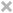Online (video chat via skype, google hangout etc)

Years of Experience in Class 12 Tuition

5

Board

CBSE

CBSE Subjects taught

Biology

Experience in School or College

it was a refreshing experience as i was open for interaction and got to learn alot in return and put my skills to the best of my ability

Taught in School or College

Yes

Reviews

this is test message this is test message this is test message this is test message this is test message this is test message this is test message

No Reviews yet! Be the first one to Review

FAQs

1. Which school boards of of Class 11 do you teach for?

CBSE

2. Have you ever taught in any School or College?

Yes

3. Which classes do you teach?

I teach BA Tuition, BCom Tuition, Class 12 Tuition, Dance and Java Training Classes.

4. Do you provide a demo class?

No, I don't provide a demo class.

5. How many years of experience do you have?

I have been teaching for 5 years.

Lessons (3)

JURISPRUDENCE: SCHOOLS OF JURISPRUDENCE

Different jurists gave different opinions regarding the nature and the meaning of law. These different views are known as Schools of Jurisprudence. 1)Sociological school: The main object of this school...

23 Jul0
Polarity of Rod in Motional EMF Electromagnetic Induction

The polarity of the RodThere is a critical topic in EMI called Motional emf. There is a specific use case where the Rod moves over between the Rails. We know that when this Rod moves in the Perpendicular...

23 Jul0
What are Kalman filters? Why they are popular in AI?

Imagine we are making a self-driving car and we are trying to localize its position in an environment. The sensors of the vehicle can detect cars, pedestrians, and cyclists. Knowing the location of these...

19 Jul0

Answers by Tasneem (1257)

Answered 4 hrs ago CBSE/Class 11/Science/Physics/Unit 7-Properties of Bulk Matter/Chapter 9-Mechanical Properties of Solids/NCERT Solutions/Exercise 9

How much should the pressure on a litre of water be changed to compress it by 0.10%?

Volume of water, V = 1 L It is given that water is to be compressed by 0.10%. Therefore, the pressure on water should be 2.2 ×106 Nm–2. ...more

Volume of water, = 1 L

It is given that water is to be compressed by 0.10%.Therefore, the pressure on water should be 2.2 ×106 Nm–2.

Dislike Bookmark

Answered 5 hrs ago CBSE/Class 11/Science/Physics/Unit 7-Properties of Bulk Matter/Chapter 10-Mechanical Properties of Fluids/NCERT Solutions/Additional Excercise 10

(a) It is known that density ρ of air decreases with height y as Where = 1.25 kg m–3 is the... ...more
(a) It is known that density ρ of air decreases with height y asWhere= 1.25 kg m–3 is the density at sea level, and y0 is a constant. This density variation is called the law of atmospheres. Obtain this law assuming that the temperature of atmosphere remains a constant (isothermal conditions). Also assume that the value of gremains constant.

(b) A large He balloon of volume 1425 m3 is used to lift a payload of 400 kg. Assume that the balloon maintains constant radius as it rises. How high does it rise?

[Take y0= 8000 m and= 0.18 kg m–3].

(a) Volume of the balloon, V = 1425 m3 Mass of the payload, m = 400 kg Acceleration due to gravity, g = 9.8 m/s2 Density of the balloon = ρ Height to which the balloon rises = y Density (ρ) of air decreases with height (y) as: This density variation is called the law of atmospherics. It can... ...more

(a) Volume of the balloon, V = 1425 m3

Mass of the payload, m = 400 kg

Acceleration due to gravity, g = 9.8 m/s2Density of the balloon = ρ

Height to which the balloon rises = y

Density (ρ) of air decreases with height (y) as:This density variation is called the law of atmospherics.

It can be inferred from equation (i) that the rate of decrease of density with height is directly proportional to ρ, i.e.,Where, k is the constant of proportionality

Height changes from 0 to y, while density changes fromto ρ.

Integrating the sides between these limits, we get:(b)Hence, the balloon will rise to a height of 8 km.

Dislike Bookmark

Answered 5 hrs ago CBSE/Class 11/Science/Physics/Unit 7-Properties of Bulk Matter/Chapter 10-Mechanical Properties of Fluids/NCERT Solutions/Additional Excercise 10

Two narrow bores of diameters 3.0 mm and 6.0 mm are joined together to form a U-tube open at both ends.... ...more
Two narrow bores of diameters 3.0 mm and 6.0 mm are joined together to form a U-tube open at both ends. If the U-tube contains water, what is the difference in its levels in the two limbs of the tube? Surface tension of water at the temperature of the experiment is 7.3 × 10–2 N m–1. Take the angle of contact to be zero and density of water to be 1.0 × 103 kg m–3 (g = 9.8 m s–2).

Diameter of the first bore, d1 = 3.0 mm = 3 × 10–3 m Diameter of the second bore, = 6.0 mm Surface tension of water, s = 7.3 × 10–2 N m–1 Angle of contact between the bore surface and water, θ= 0 Density of water, ρ =1.0 × 103 kg/m–3 Acceleration... ...more

Diameter of the first bore, d1 = 3.0 mm = 3 × 10–3 mDiameter of the second bore,= 6.0 mmSurface tension of water, s = 7.3 × 10–2 N m–1

Angle of contact between the bore surface and water, θ= 0

Density of water, ρ =1.0 × 103 kg/m–3

Acceleration due to gravity, g = 9.8 m/s2

Let h1 and hbe the heights to which water rises in the first and second tubes respectively. These heights are given by the relations:The difference between the levels of water in the two limbs of the tube can be calculated as:Hence, the difference between levels of water in the two bores is 4.97 mm.

Dislike Bookmark

Answered 5 hrs ago CBSE/Class 11/Science/Physics/Unit 7-Properties of Bulk Matter/Chapter 10-Mechanical Properties of Fluids/NCERT Solutions/Additional Excercise 10

Mercury has an angle of contact equal to 140° with soda lime glass. A narrow tube of radius 1.00... ...more
Mercury has an angle of contact equal to 140° with soda lime glass. A narrow tube of radius 1.00 mm made of this glass is dipped in a trough containing mercury. By what amount does the mercury dip down in the tube relative to the liquid surface outside? Surface tension of mercury at the temperature of the experiment is 0.465 N m–1. Density of mercury = 13.6 × 103 kg m–3.

Angle of contact between mercury and soda lime glass, θ = 140° Radius of the narrow tube, r = 1 mm = 1 × 10–3 m Surface tension of mercury at the given temperature, s = 0.465 N m–1 Density of mercury, ρ =13.6 × 103 kg/m3 Dip in the height of mercury = h Acceleration... ...more

Angle of contact between mercury and soda lime glass, θ = 140°

Radius of the narrow tube, r = 1 mm = 1 × 10–3 m

Surface tension of mercury at the given temperature, s = 0.465 N m–1

Density of mercury, ρ =13.6 × 103 kg/m3

Dip in the height of mercury = h

Acceleration due to gravity, g = 9.8 m/s2

Surface tension is related with the angle of contact and the dip in the height as:Here, the negative sign shows the decreasing level of mercury. Hence, the mercury level dips by 5.34 mm.

Dislike Bookmark

Answered 5 hrs ago CBSE/Class 11/Science/Physics/Unit 7-Properties of Bulk Matter/Chapter 10-Mechanical Properties of Fluids/NCERT Solutions/Additional Excercise 10

In Millikan’s oil drop experiment, what is the terminal speed of an uncharged drop of radius 2.0... ...more
In Millikan’s oil drop experiment, what is the terminal speed of an uncharged drop of radius 2.0 × 10–5 m and density 1.2 × 103 kg m–3? Take the viscosity of air at the temperature of the experiment to be 1.8 × 10–5 Pa s. How much is the viscous force on the drop at that speed? Neglect buoyancy of the drop due to air.

Terminal speed = 5.8 cm/s; Viscous force = 3.9 × 10–10 N Radius of the given uncharged drop, r = 2.0 × 10–5 m Density of the uncharged drop, ρ = 1.2 × 103 kg m–3 Viscosity of air, Density of air can be taken as zero in order to neglect buoyancy of air. Acceleration... ...more

Terminal speed = 5.8 cm/s; Viscous force = 3.9 × 10–10 N

Radius of the given uncharged drop, r = 2.0 × 10–5 m

Density of the uncharged drop, ρ = 1.2 × 103 kg m–3

Viscosity of air,Density of aircan be taken as zero in order to neglect buoyancy of air.

Acceleration due to gravity, g = 9.8 m/s2

Terminal velocity (v) is given by the relation:Hence, the terminal speed of the drop is 5.8 cm s–1.

The viscous force on the drop is given by:Hence, the viscous force on the drop is 3.9 × 10–10 N.

Dislike Bookmark

TasneemDirections

x
Class 12 Tuition 3.8

Class LocationStudent's HomeTutor's HomeOnline (video chat via skype, google hangout etc)

Years of Experience in Class 12 Tuition

5

Board

CBSE

CBSE Subjects taught

Biology

Experience in School or College

it was a refreshing experience as i was open for interaction and got to learn alot in return and put my skills to the best of my ability

Taught in School or College

Yes

BCom Tuition 3.4

Class LocationStudent's HomeTutor's HomeOnline (video chat via skype, google hangout etc)

Years of Experience in BCom Tuition

5

BCom Subject

Business Mathematics and Statistics, Business Ethics, Organisational Behaviour

Experience in School or College

it was thrilling

Type of class

Regular Classes

Class strength catered to

One on one/ Private Tutions

Taught in School or College

Yes

BA Tuition 3.4

Class LocationStudent's HomeTutor's HomeOnline (video chat via skype, google hangout etc)

Years of Experience in BA Tuition

5

Field tutored for

Bio-Chemistry, Micro-Biology

Experience in School or College

it was thrilling

Type of class

Crash Course, Regular Classes

Class strength catered to

Group Classes

BA Bio-Chemistry Subjects

Proteins, Metabolism of Amino Acids and Nucleotides, Cell Biology, Gene Organization, Replication and Repair, Metabolism of Carbohydrates and Lipids, Human Physiology, Enzymes

Taught in School or College

Yes

BA Micro- Biology Subjects

Biochemistry, Microbial Genetics And Genomics, Molecular Biology, Bacteriology

Dance Classes 2.8

Class LocationStudent's HomeTutor's HomeOnline (video chat via skype, google hangout etc)

Java Training Classes 2.8

Class LocationStudent's HomeTutor's HomeOnline (video chat via skype, google hangout etc)

this is test message this is test message this is test message this is test message this is test message this is test message this is test message

No Reviews yet! Be the first one to Review

Answers by Tasneem (1257)

Answered 4 hrs ago CBSE/Class 11/Science/Physics/Unit 7-Properties of Bulk Matter/Chapter 9-Mechanical Properties of Solids/NCERT Solutions/Exercise 9

How much should the pressure on a litre of water be changed to compress it by 0.10%?

Volume of water, V = 1 L It is given that water is to be compressed by 0.10%. Therefore, the pressure on water should be 2.2 ×106 Nm–2. ...more

Volume of water, = 1 L

It is given that water is to be compressed by 0.10%.Therefore, the pressure on water should be 2.2 ×106 Nm–2.

Dislike Bookmark

Answered 5 hrs ago CBSE/Class 11/Science/Physics/Unit 7-Properties of Bulk Matter/Chapter 10-Mechanical Properties of Fluids/NCERT Solutions/Additional Excercise 10

(a) It is known that density ρ of air decreases with height y as Where = 1.25 kg m–3 is the... ...more
(a) It is known that density ρ of air decreases with height y asWhere= 1.25 kg m–3 is the density at sea level, and y0 is a constant. This density variation is called the law of atmospheres. Obtain this law assuming that the temperature of atmosphere remains a constant (isothermal conditions). Also assume that the value of gremains constant.

(b) A large He balloon of volume 1425 m3 is used to lift a payload of 400 kg. Assume that the balloon maintains constant radius as it rises. How high does it rise?

[Take y0= 8000 m and= 0.18 kg m–3].

(a) Volume of the balloon, V = 1425 m3 Mass of the payload, m = 400 kg Acceleration due to gravity, g = 9.8 m/s2 Density of the balloon = ρ Height to which the balloon rises = y Density (ρ) of air decreases with height (y) as: This density variation is called the law of atmospherics. It can... ...more

(a) Volume of the balloon, V = 1425 m3

Mass of the payload, m = 400 kg

Acceleration due to gravity, g = 9.8 m/s2Density of the balloon = ρ

Height to which the balloon rises = y

Density (ρ) of air decreases with height (y) as:This density variation is called the law of atmospherics.

It can be inferred from equation (i) that the rate of decrease of density with height is directly proportional to ρ, i.e.,Where, k is the constant of proportionality

Height changes from 0 to y, while density changes fromto ρ.

Integrating the sides between these limits, we get:(b)Hence, the balloon will rise to a height of 8 km.

Dislike Bookmark

Answered 5 hrs ago CBSE/Class 11/Science/Physics/Unit 7-Properties of Bulk Matter/Chapter 10-Mechanical Properties of Fluids/NCERT Solutions/Additional Excercise 10

Two narrow bores of diameters 3.0 mm and 6.0 mm are joined together to form a U-tube open at both ends.... ...more
Two narrow bores of diameters 3.0 mm and 6.0 mm are joined together to form a U-tube open at both ends. If the U-tube contains water, what is the difference in its levels in the two limbs of the tube? Surface tension of water at the temperature of the experiment is 7.3 × 10–2 N m–1. Take the angle of contact to be zero and density of water to be 1.0 × 103 kg m–3 (g = 9.8 m s–2).

Diameter of the first bore, d1 = 3.0 mm = 3 × 10–3 m Diameter of the second bore, = 6.0 mm Surface tension of water, s = 7.3 × 10–2 N m–1 Angle of contact between the bore surface and water, θ= 0 Density of water, ρ =1.0 × 103 kg/m–3 Acceleration... ...more

Diameter of the first bore, d1 = 3.0 mm = 3 × 10–3 mDiameter of the second bore,= 6.0 mmSurface tension of water, s = 7.3 × 10–2 N m–1

Angle of contact between the bore surface and water, θ= 0

Density of water, ρ =1.0 × 103 kg/m–3

Acceleration due to gravity, g = 9.8 m/s2

Let h1 and hbe the heights to which water rises in the first and second tubes respectively. These heights are given by the relations:The difference between the levels of water in the two limbs of the tube can be calculated as:Hence, the difference between levels of water in the two bores is 4.97 mm.

Dislike Bookmark

Answered 5 hrs ago CBSE/Class 11/Science/Physics/Unit 7-Properties of Bulk Matter/Chapter 10-Mechanical Properties of Fluids/NCERT Solutions/Additional Excercise 10

Mercury has an angle of contact equal to 140° with soda lime glass. A narrow tube of radius 1.00... ...more
Mercury has an angle of contact equal to 140° with soda lime glass. A narrow tube of radius 1.00 mm made of this glass is dipped in a trough containing mercury. By what amount does the mercury dip down in the tube relative to the liquid surface outside? Surface tension of mercury at the temperature of the experiment is 0.465 N m–1. Density of mercury = 13.6 × 103 kg m–3.

Angle of contact between mercury and soda lime glass, θ = 140° Radius of the narrow tube, r = 1 mm = 1 × 10–3 m Surface tension of mercury at the given temperature, s = 0.465 N m–1 Density of mercury, ρ =13.6 × 103 kg/m3 Dip in the height of mercury = h Acceleration... ...more

Angle of contact between mercury and soda lime glass, θ = 140°

Radius of the narrow tube, r = 1 mm = 1 × 10–3 m

Surface tension of mercury at the given temperature, s = 0.465 N m–1

Density of mercury, ρ =13.6 × 103 kg/m3

Dip in the height of mercury = h

Acceleration due to gravity, g = 9.8 m/s2

Surface tension is related with the angle of contact and the dip in the height as:Here, the negative sign shows the decreasing level of mercury. Hence, the mercury level dips by 5.34 mm.

Dislike Bookmark

Answered 5 hrs ago CBSE/Class 11/Science/Physics/Unit 7-Properties of Bulk Matter/Chapter 10-Mechanical Properties of Fluids/NCERT Solutions/Additional Excercise 10

In Millikan’s oil drop experiment, what is the terminal speed of an uncharged drop of radius 2.0... ...more
In Millikan’s oil drop experiment, what is the terminal speed of an uncharged drop of radius 2.0 × 10–5 m and density 1.2 × 103 kg m–3? Take the viscosity of air at the temperature of the experiment to be 1.8 × 10–5 Pa s. How much is the viscous force on the drop at that speed? Neglect buoyancy of the drop due to air.

Terminal speed = 5.8 cm/s; Viscous force = 3.9 × 10–10 N Radius of the given uncharged drop, r = 2.0 × 10–5 m Density of the uncharged drop, ρ = 1.2 × 103 kg m–3 Viscosity of air, Density of air can be taken as zero in order to neglect buoyancy of air. Acceleration... ...more

Terminal speed = 5.8 cm/s; Viscous force = 3.9 × 10–10 N

Radius of the given uncharged drop, r = 2.0 × 10–5 m

Density of the uncharged drop, ρ = 1.2 × 103 kg m–3

Viscosity of air,Density of aircan be taken as zero in order to neglect buoyancy of air.

Acceleration due to gravity, g = 9.8 m/s2

Terminal velocity (v) is given by the relation:Hence, the terminal speed of the drop is 5.8 cm s–1.

The viscous force on the drop is given by:Hence, the viscous force on the drop is 3.9 × 10–10 N.

Dislike Bookmark

Lessons (3)

JURISPRUDENCE: SCHOOLS OF JURISPRUDENCE

Different jurists gave different opinions regarding the nature and the meaning of law. These different views are known as Schools of Jurisprudence. 1)Sociological school: The main object of this school...

23 Jul0
Polarity of Rod in Motional EMF Electromagnetic Induction

The polarity of the RodThere is a critical topic in EMI called Motional emf. There is a specific use case where the Rod moves over between the Rails. We know that when this Rod moves in the Perpendicular...

23 Jul0
What are Kalman filters? Why they are popular in AI?

Imagine we are making a self-driving car and we are trying to localize its position in an environment. The sensors of the vehicle can detect cars, pedestrians, and cyclists. Knowing the location of these...

19 Jul0

Tasneem conducts classes in BA Tuition, BCom Tuition and Class 12 Tuition. Tasneem is located in Arekere, Bangalore. Tasneem takes at students Home and Regular Classes- at her Home. She has 5 years of teaching experience . Tasneem has completed Bachelor of Computer Science (B.Sc. (Computer Science)) from the oxford college of dental in 2010. She is well versed in Hindi, Tamil and English.

Recommended Profiles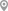Madiwala, BangaloreDevasandra, BangaloreKoramangala 1st Block, BangaloreJ P Nagar, BangaloreChamrajpet, BangaloreMathikere, Bangalore

X

Post your Learning Need

Let us shortlist and give the best tutors and institutes.

or

Send Enquiry to Tasneem

Let Tasneem know you are interested in their class

Reply to 's review